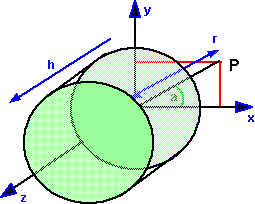# Maths - Cylindrical Polar coordinates

 Cylindrical coordinates allow points to be specified using two linear distances and one angle. These three coordinates are shown on the diagram as: r = radius (distance from axis of cylinder) h = height (distance along axis of cylinder) θ = (sometimes a) = angle around axis.## Cylinder aligned with the z axis

### Cylindrical coordinates to Cartesian coordinates

 Cx Cy Cz
=
 r sin(θ) r cos(θ) h

### Cartesian coordinates to Cylindrical coordinates

 θ r h
=
 atan2(Cx,Cy) √(Cx2 + Cy2) Cz

## Cylinder aligned with the arbitrary axis

### Cylindrical coordinates to Cartesian coordinates

We want to rotate the above so that the h axis is aligned with the arbitrary axis (Ax, Ay, Az) in other words we want to lookat the point (Ax, Ay, Az) see lookat

 Cx Cy Cz
=
 √(Az2 + Ay2) 0 Ax 0 √(Az2 + Ax2) Ay -Ax -Ay Az
 r sin(θ) r cos(θ) h

Multiplying gives:

 Cx Cy Cz
=
 √(Az2 + Ay2) * r sin(θ) + h*Ax √(Az2 + Ax2) * r cos(θ) + h*Ay -Ax * r sin(θ)-Ay * r cos(θ) + h*Az

### Cartesian coordinates to Cylindrical coordinates

In order to do this we need to invert the above matrix, since this is orthogonal we can invert by transposing as follows:

 r sin(θ) r cos(θ) h
=
 √(Az2 + Ay2) 0 -Ax 0 √(Az2 + Ax2) -Ay Ax Ay Az
 Cx Cy Cz

Multiplying gives,

 r sin(θ) r cos(θ) h
=
 Cx * √(Az2 + Ay2) - Ax * Cz Cy * √(Az2 + Ax2) -Ay * Cz Cx * Ax + Cy * Ay +Cz * Az

So in terms of θ, r , h

 θ r h
=
 atan2(Cx * √(Az2 + Ay2) - Ax * Cz,Cy * √(Az2 + Ax2) -Ay * Cz) √((Cx * √(Az2 + Ay2) - Ax * Cz)2 + (Cy * √(Az2 + Ax2) -Ay * Cz)2) Cx * Ax + Cy * Ay +Cz * Az

## Using Tensors

This is more advanced stuff and only really needed if you need to do physics or advanced geometry in curvilinear coordinates.

On the curvilinear coordinates page we saw that the expression of coordinates as a linear equation:

ei Ai = e1 A1 + e2 A2 + e3 A3

can be modified for curvilinear coordinates where either the basis or the components depend on the location:

e(x,y,z)i Ai = e(x,y,z)1 A1 + e(x,y,z)2 A2 + e(x,y,z)3 A3

or

ei A(x,y,z)i = e1 A(x,y,z)1 + e2 A(x,y,z)2 + e3 A(x,y,z)3

where:

• e(x,y,z) is a basis which is a function of position.
• A(x,y,z) are terms which are functions of position.

We have already looked at the situation where the terms are expressed as a function of a global linear coordinate system. Now lets look at the situation where the linear basis is a function of position. There are two ways to do this:

• they can be built along the coordinate axes (axis-colinear) then the basis vectors transform like covariant vectors.
• they can be built to be perpendicular (normal) to the coordinate surfaces then the basis vectors transform like contravariant vectors.

### Covariant Axis

 e1 A1 + e2 A2 + e3 A3 = ∂x e1 ∂θ A1 + ∂y e2 ∂r A2 + ∂z e3 ∂h A3 ∂θ ∂x ∂r ∂y ∂h ∂z

where:

 ∂θ = ∂atan2(x,y) = y = y ∂x ∂x 1 +(x/y)2 x2+ y 2 ∂r = √((x)2) + (y)2) = 1 ∂y ∂y ∂h = 1 ∂z

where:

 ∂ = cosθ ∂ -r-1sinθ ∂ ∂x ∂r ∂θ ∂ = sinθ ∂ +r-1cosθ ∂ ∂y ∂r ∂θ ∂ = ∂ ∂z ∂h

Jacobean

J =
 ∂x'i ∂xj
=
 x √(x2 + y2)
 y √(x2 + y2)
0
 -y (x2 + y2)
 x (x2 + y2)
0
0 0 1
=
 cosθ sinθ 0 -r-1sinθ r-1cosθ 0 0 0 1

Inverse Jacobean

J-1 =
 ∂xj ∂x'i
=
 x √(x2 + y2)
-y 0
 -y (x2 + y2)
x 0
0 0 1
=
 cosθ sinθ 0 r sinθ r cosθ 0 0 0 1

### Contravariant Axis

 e1 A1 + e2 A2 + e3 A3 = ∂θ e1 ∂x1 A1 + ∂r e2 ∂x2 A2 + ∂h e3 ∂x3 A3 ∂x1 ∂θ ∂x2 ∂r ∂x3 ∂h